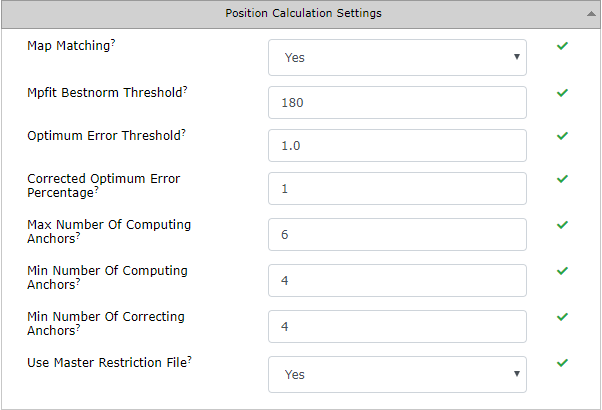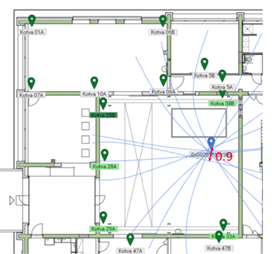# Position Calculation Settings## Map Matching

possible values:  Yes / No

default: Yes

Globally enables/disables map matching functionality.

## Mpfit Bestnorm Threshold

possible values:  number in range from 0 to 10 000

default: 180

This is one of parameters that evaluate precision of computed position. Lower values (<100) means that result of calculation is stricter in terms of calculation error. However, this does not mean that position alone is more precise. Higher values (>300) relax calculation error threshold.

## Optimum Error Threshold

possible values:  range from 0 to 1000 [m]

default: 1

Primary parameter used for calculation evaluation. Calculated position is intersection of multiple hyperbolas formed by anchors. If farthest distance between found optimum and one of hyperbolas is worse than this parameter’s value, then the recalculation is initiated.Worst distance between found optimum and each hyperbola is 0.9m.
That is below threshold, therefore no recalculation is initiated.

## Corrected Optimum Threshold

possible values: range from 0 to 1000 [%]

default: 1

Evaluation parameter of recalculation like Optimum Error Threshold – still measured by worst distance from optimum to hyperbolas. However, for recalculation the constraints need to be relaxed in order to retrieve more results. This threshold is set dynamically according to the size perimeter formed by anchors.

For example, localization area is 400 m2, then 1% is 4 and this value is used as threshold.

## Max Number Of Computing Anchors

possible values: number in range from 3 to 100

default: 6

Maximum number of anchors used for calculation. In practice, in indoor positioning tag usually has unobstructed vision to 4-6 anchors, others are non-line-of-sight that might worsen the results. Therefore, the default value is 6. If there is good signal from more than 6 anchors, RTLS Server tries to select the best six (in terms of signal, synchronization, geometry, etc.)  to calculate position.

## Min Number Of Computing Anchors

possible values: number in range from 3 to 100

default: 4

Minimum number of anchors used for calculation. Mathematically, minimum is 3, however 4th anchor adds redundancy and ability to calculate the error of computation.

## Min Number Of Correcting Anchors

possible values: number in range from 3 to 100

default: 4

Minimum number of anchors used for recalculation.

## Use Master Restriction File

possible values:  Yes / No

default: Yes

Restrict localization to certain anchors in certain areas. More details is in Anchor Restrictions